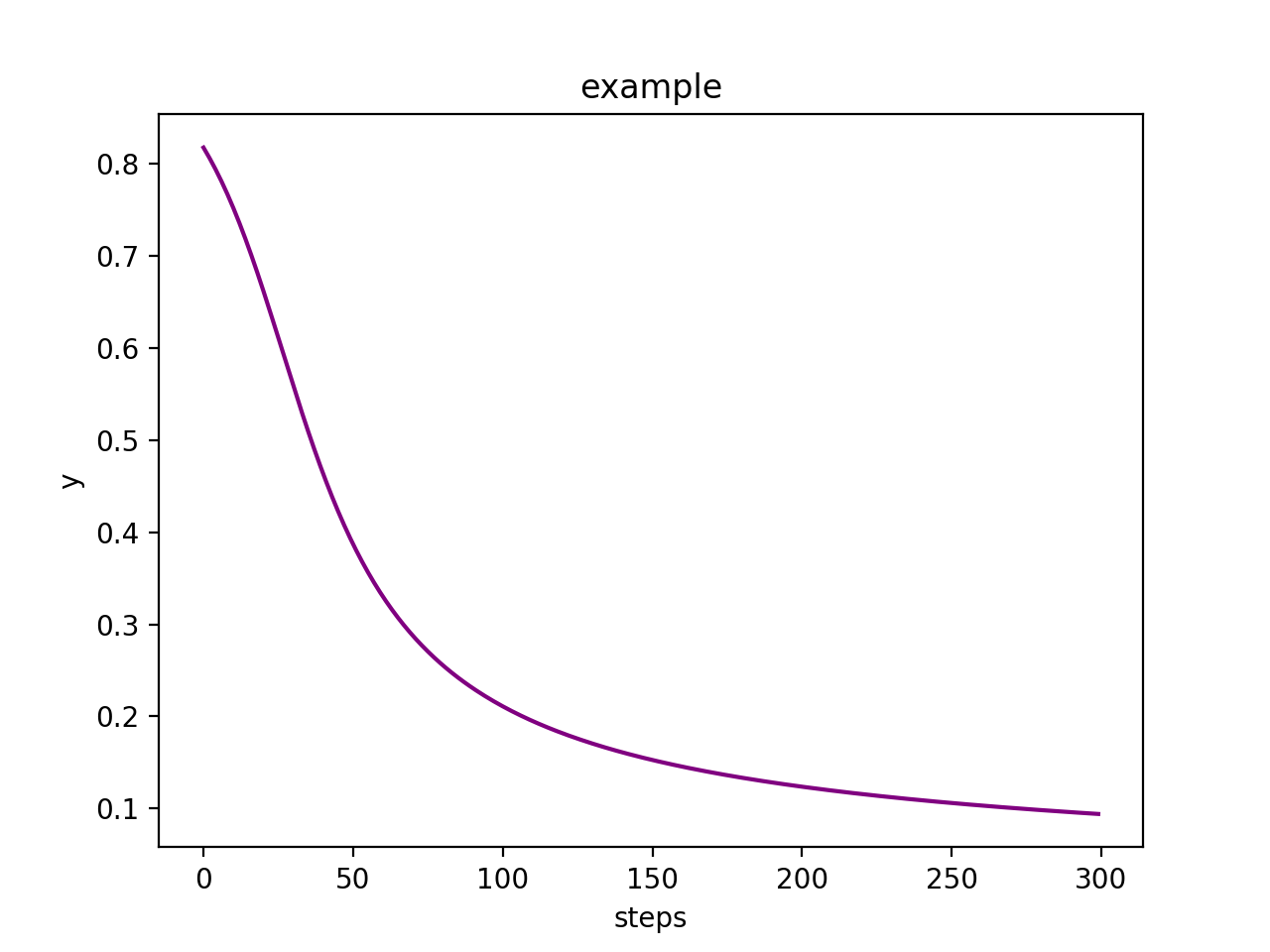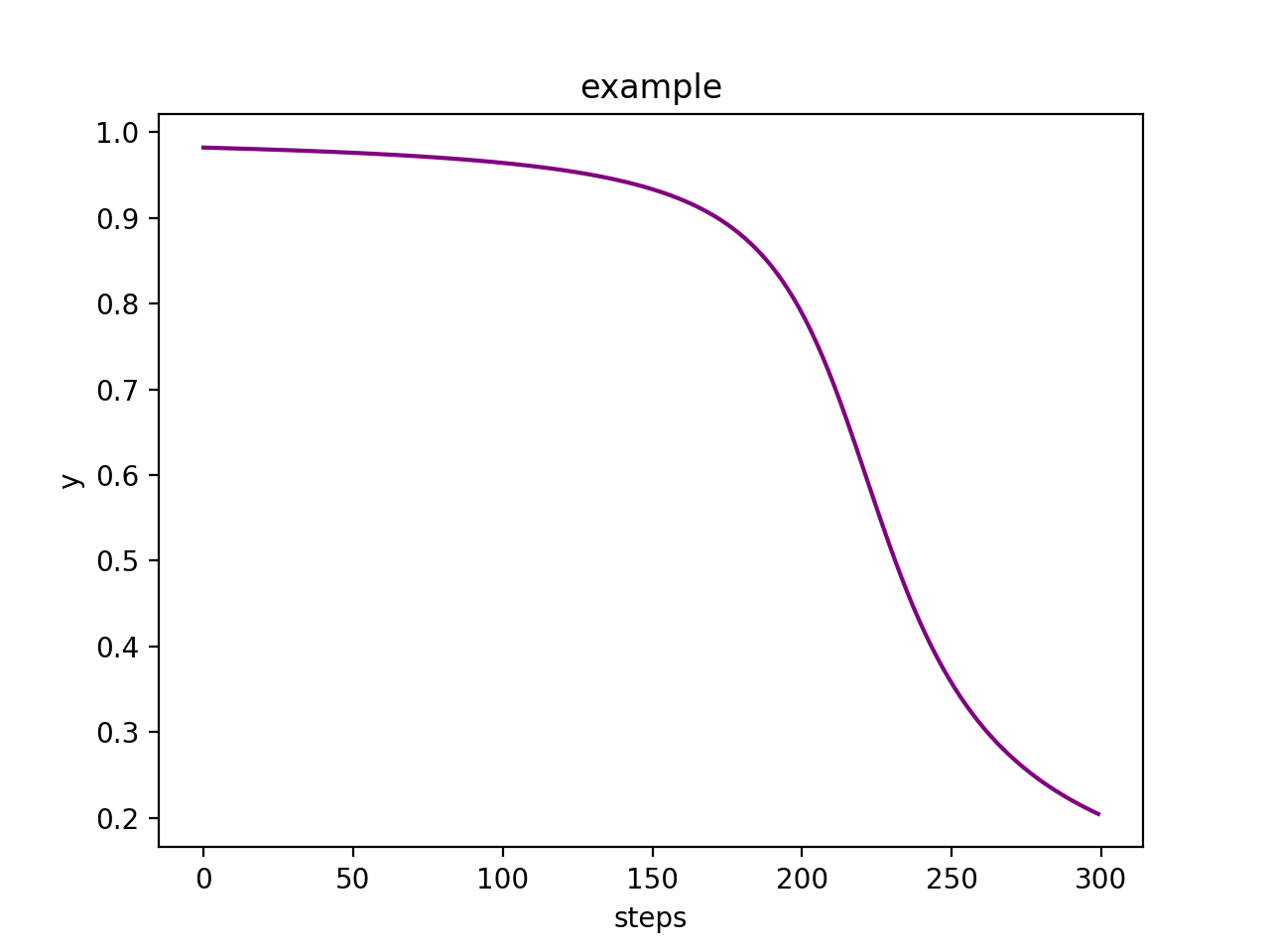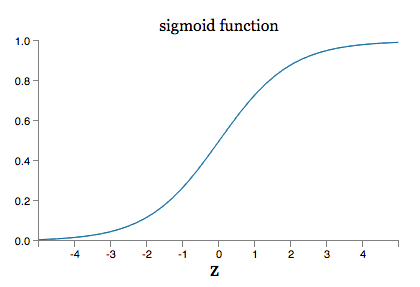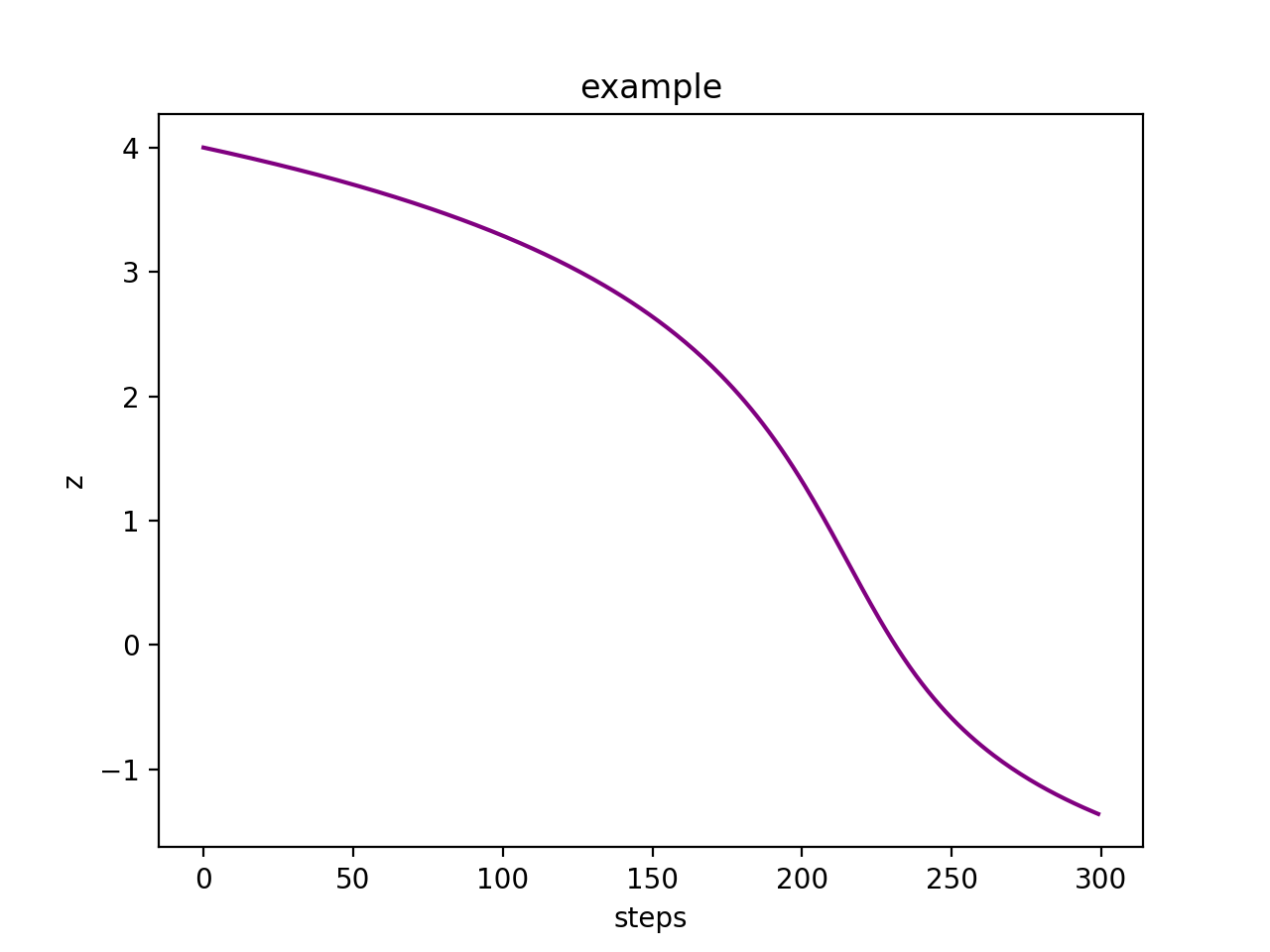深度神经网络调参之损失函数

TED有这样一个演讲How to learn from mistakes,演讲者主要分享了一些学习的体会，其中最主要的就是如何从自己犯过的错误中学习。我们自己学习工作中应该很有体会，踩过的坑下次就知道了。相反如果学习过程中一点错误都没用，那就需要担心自己的学习效率了。

梯度消失import tensorflow as tf
xs = tf.placeholder(tf.float32)
ys = tf.placeholder(tf.float32)

w = tf.Variable(tf.constant(0.6))
b = tf.Variable(tf.constant(0.9))

y = tf.nn.sigmoid(w*xs+b)
loss = tf.pow(ys-y, 2)/2
sess = tf.Session()
# important step
sess.run(tf.initialize_all_variables())
for i in range(300):
_,y_o = sess.run([train_step,y], feed_dict={xs: 1, ys: 0})
print(y_o)$\begin{array}{}\text{(1)}& C=\frac{\left(y-a{\right)}^{2}}{2}\end{array}$

$x$$x$表示输入，$z$$z$表示隐藏神经元的输入，则有$z=wx+b$$z = wx+b$通过激活函数后则有$a=\sigma \left(z\right)$$a =\sigma(z)$，不难求得

$\begin{array}{}\text{(2)}& \frac{\mathrm{\partial }C}{\mathrm{\partial }w}& =& \left(a-y\right){\sigma }^{\prime }\left(z\right)x\text{(3)}& \frac{\mathrm{\partial }C}{\mathrm{\partial }b}& =& \left(a-y\right){\sigma }^{\prime }\left(z\right)\end{array}$交叉熵损失函数

$C=-\frac{1}{n}\sum _{i}{y}_{i}\mathrm{ln}{a}_{i}$

${a}_{i}$$a_i$是模型的输出，表示当前输入被分为第i类的概率
${y}_{i}$$y_i$是当前的输入对应的label(是第i类为1，否则为0)

$C=-\frac{1}{n}\left[y\mathrm{ln}a+\left(1-y\right)\mathrm{ln}\left(1-a\right)\right]$

$C=-\left[y\mathrm{ln}a+\left(1-y\right)\mathrm{ln}\left(1-a\right)\right]$

$\begin{array}{}\text{(1)}& \frac{\mathrm{\partial }C}{\mathrm{\partial }w}& =& \left(a-y\right)x\text{(2)}& \frac{\mathrm{\partial }C}{\mathrm{\partial }b}& =& \left(a-y\right)\end{array}$

loss = -tf.reduce_mean(ys*tf.log(y))